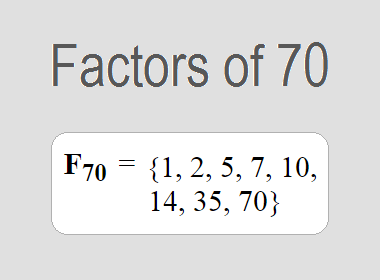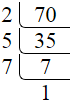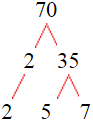# Factors of 70The factors of 70 are 1, 2, 5, 7, 10, 14, 35, and 70 i.e. F70 = {1, 2, 5, 7, 10, 14, 35, 70}. The factors of 70 are those numbers that can divide 70 without leaving a remainder.

We can check if these numbers are factors of 70 by dividing 70 by each of them. If the result is a whole number, then the number is a factor of 70. Let's do this for each of the numbers listed above:

·        1 is a factor of 70 because 70 divided by 1 is 70.

·        2 is a factor of 70 because 70 divided by 2 is 35.

·        5 is a factor of 70 because 70 divided by 5 is 14.

·        7 is a factor of 70 because 70 divided by 7 is 10.

·        10 is a factor of 70 because 70 divided by 10 is 7.

·        14 is a factor of 70 because 70 divided by 14 is 5.

·        35 is a factor of 70 because 70 divided by 35 is 2.

·        70 is a factor of 70 because 70 divided by 70 is 1.

## How to Find Factors of 70?

1 and the number itself are the factors of every number. So, 1 and 70 are two factors of 70. To find the other factors of 70, we can start by dividing 70 by the numbers between 1 and 70. If we divide 70 by 2, we get a remainder of 0. Therefore, 2 is a factor of 70. If we divide 70 by 3, we get a remainder of 1. Therefore, 3 is not a factor of 70.

Next, we can check if 4 is a factor of 70. If we divide 70 by 4, we get a remainder of 2. Therefore, 4 is not a factor of 70. If we divide 70 by 5, we get a remainder of 0. Therefore, 5 is also a factor of 70. We can continue this process for all the possible factors of 70.

Through this process, we can find that the factors of 70 are 1, 2, 5, 7, 10, 14, 35, and 70. These are the only numbers that can divide 70 without leaving a remainder.

********************

********************

## Properties of the Factors of 70

The factors of 70 have some interesting properties. One of the properties is that the sum of the factors of 70 is equal to 144. We can see this by adding all the factors of 70 together:

1 + 2 + 5 + 7 + 10 + 14 + 35 + 70 = 144

Another property of the factors of 70 is that the prime factors of 70 are 2, 5, and 7.

## Applications of the Factors of 70

The factors of 70 have several applications in mathematics. One of the applications is in finding the highest common factor (HCF) of two or more numbers. The HCF is the largest factor that two or more numbers have in common. For example, to find the HCF of 70 and 80, we need to find the factors of both numbers and identify the largest factor they have in common. The factors of 70 are 1, 2, 5, 7, 10, 14, 35, and 70. The factors of 80 are 1, 2, 4, 5, 8, 10, 16, 20, 40, and 80. The largest factor that they have in common is 10. Therefore, the HCF of 70 and 80 is 10.

Another application of the factors of 70 is in prime factorization. Prime factorization is the process of expressing a number as the product of its prime factors. The prime factors of 70 are 2, 5, and 7, since these are the only prime numbers that can divide 70 without leaving a remainder. Therefore, we can express 70 as:

70 = 2 × 5 × 7

We can do prime factorization by division and factor tree method also. Here is the prime factorization of 70 by division method,70 = 2 × 5 × 7

Here is the prime factorization of 70 by the factor tree method,70 = 2 × 5 × 7## Conclusion

The factors of 70 are the numbers that can divide 70 without leaving a remainder. The factors of 70 are 1, 2, 5, 7, 10, 14, 35, and 70. The factors of 70 have some interesting properties, such as having a sum of 144. The factors of 70 have several applications in mathematics, such as finding the highest common factor and prime factorization.